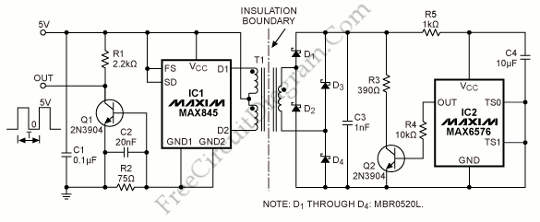## AD596/597 Setpoint Control for Temperature Controller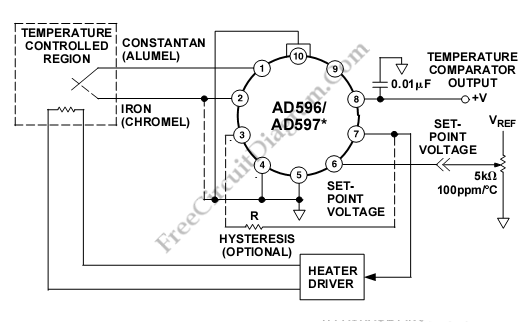This is a setpoint controller circuit using The AD596/AD597. The thermocouple voltage is amplified, compared to an external setpoint voltage, and cold junction compensated. The device temperature can be monitored if the temperature to be controlled is within the operating range (–55°C to +125°C) of the device. Here is the schematic diagram of the circuit: Setpoint Voltage of AD597 is  […]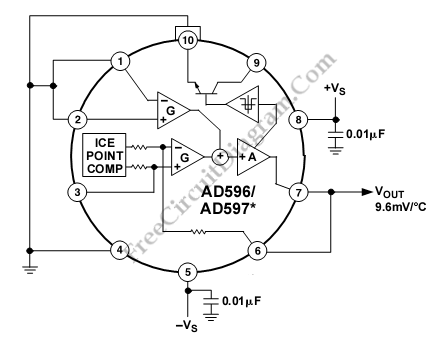This is a stand alone temperature transducer/sensor circuit. This device uses The AD596/AD597, employing its internal junction compensation temperature sensor inside. This device can be used as temperature sensor by omitting the thermocouple and connecting the inputs (Pins 1 and 2) to common. Here is the schematic diagram of the circuit: The output will reflect the compensation voltage and the […]

## Linearized Platinum RTD Signal Conditioner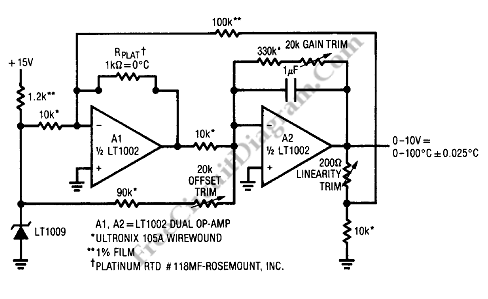The best choice for stability and high accuracy in temperature measurements is Platinum resistance temperature detectors (RTD). However, the signal conditioning is complicated by the RTD which exhibit a non-linear temperature versus resistance characteristic. The non-linearity amount of 0 C to 100 C is up to 0.4 C. Here is the schematic diagram of linearized platinum RTD signal conditioner : […]

## Board System Monitoring for Temperature and Voltage Condition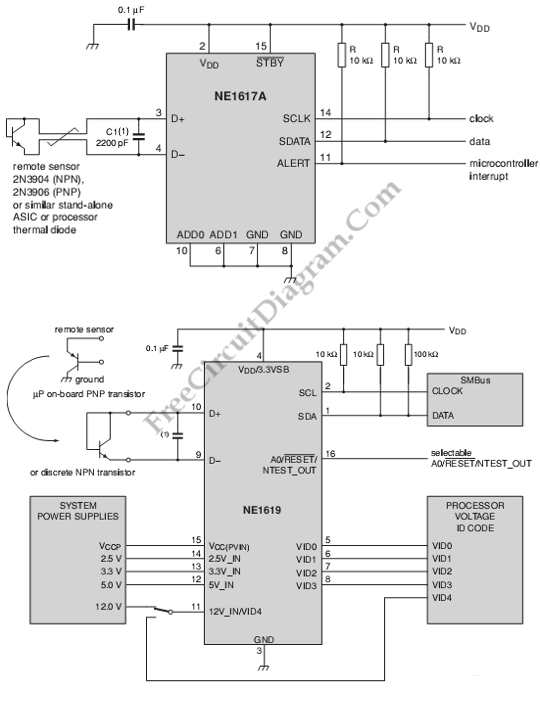This is a circuit of board system monitoring for temperature and voltage condition. It uses the NE1617A. The NE1617A is a 2 channel temperature sensor. It can measure remote and internal sensor. It also supports up to nine devices per bus. The temperature data update is selectable from 125 ms to 16 seconds, if it is used in normal operation. […]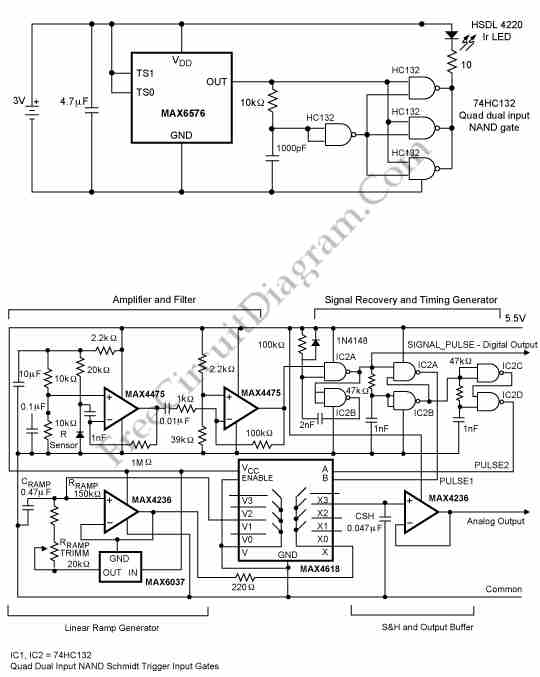This is a circuit that transmits temperature data over an IR link and it allows the isolation of temperature sensor. It is a good solution of obtaining temperature readings from points at potentials a few tens of volts from ground by linking a temperature probe  only by an IR connection to the readout point. This circuit consist of a temperature […]

## Analog Compensation Circuit for PT100 RTD Temperature Sensor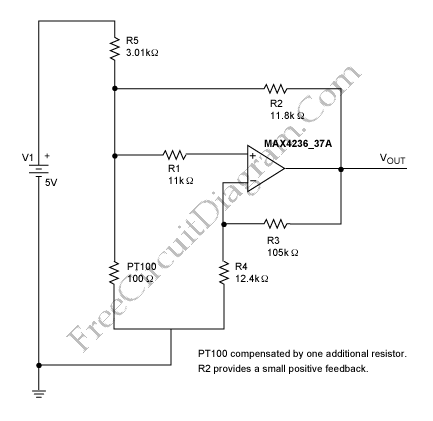There are some digital and analog that can be used for compensating a PT100 RTD nonlinearity. We can implemented the digital linearization by implementing the previous generic equation  or by a lookup table. By implementing the previous generic equation we can calculate temperature values directly, based on the actual measured RTD resistance. A lookup table allows an application to convert […]